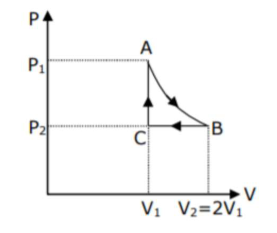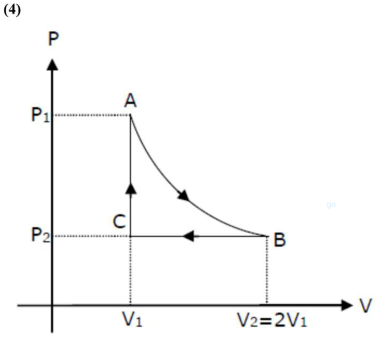# n mole of a perfect gas undergoes a cyclic process ABCA (see figure) consisting of the following processes -Question:

$\mathrm{n}$ mole of a perfect gas undergoes a cyclic process $\mathrm{ABCA}$ (see figure) consisting of the following processes -

$\mathrm{A} \rightarrow \mathrm{B}$ : Isothermal expansion at temperature $\mathrm{T}$ so that the volume is doubled from $\mathrm{V}_{1}$ to $\mathrm{V}_{2}=2 \mathrm{~V}_{1}$ and pressure changes from $\mathrm{P}_{1}$ to $\mathrm{P}_{2}$. $\mathrm{B} \rightarrow \mathrm{C}$ : Isobaric compression at pressure $\mathrm{P}_{2}$ to initial volume $\mathrm{V}_{1}$. $\mathrm{C} \rightarrow \mathrm{A}$ : Isochoric change leading to change of pressure from $\mathrm{P}_{2}$ to $\mathrm{P}_{1}$. Total workdone in the complete cycle $\mathrm{ABCA}$ is -1. (1) 0

2. 2) nRT $\left(\ln 2+\frac{1}{2}\right)$

3. (3) nRTIn2

4. (4) $\mathrm{nRT}\left(\ln 2-\frac{1}{2}\right)$

Correct Option: 4,

Solution:$A \rightarrow B=$ isotheraml process

$\mathrm{B} \rightarrow \mathrm{C}=$ isobaric process

$C \rightarrow A=$ isochoric process

also, $V_{2}=2 V_{1}$

work done by gas in the complete cycle $\mathrm{ABCA}$ is